Rotational Symmetry

Chapter 14 Class 7 Symmetry
Concept wise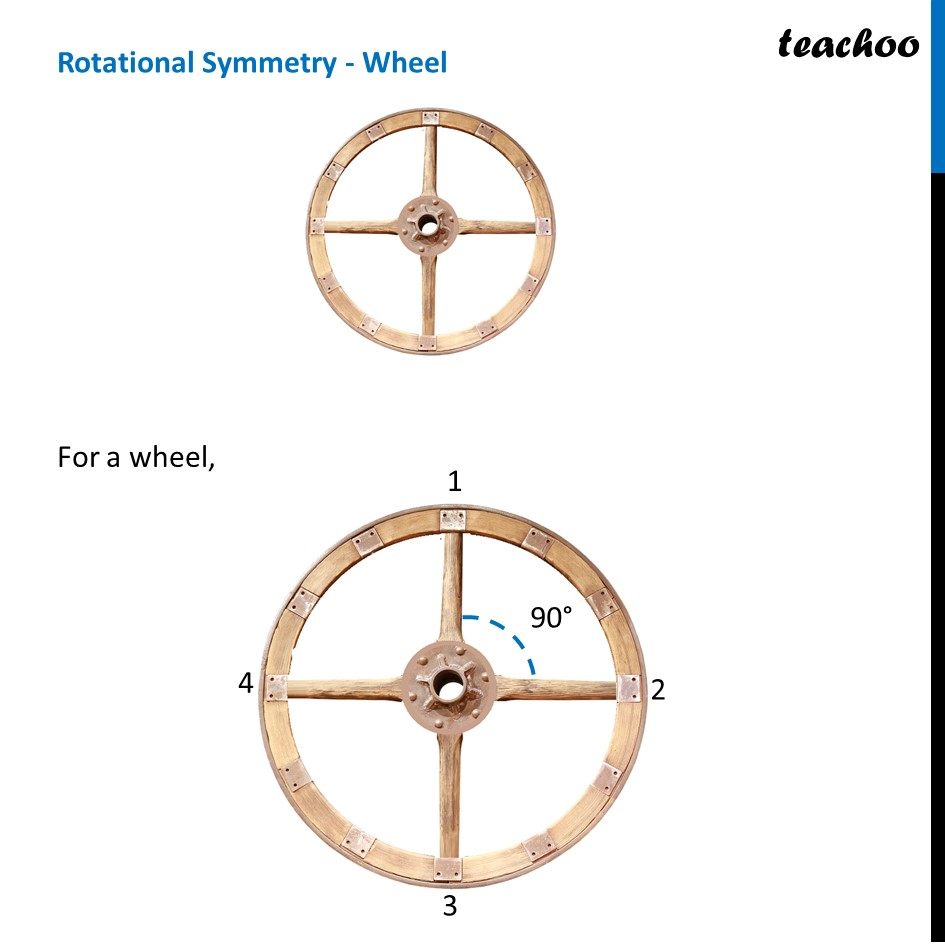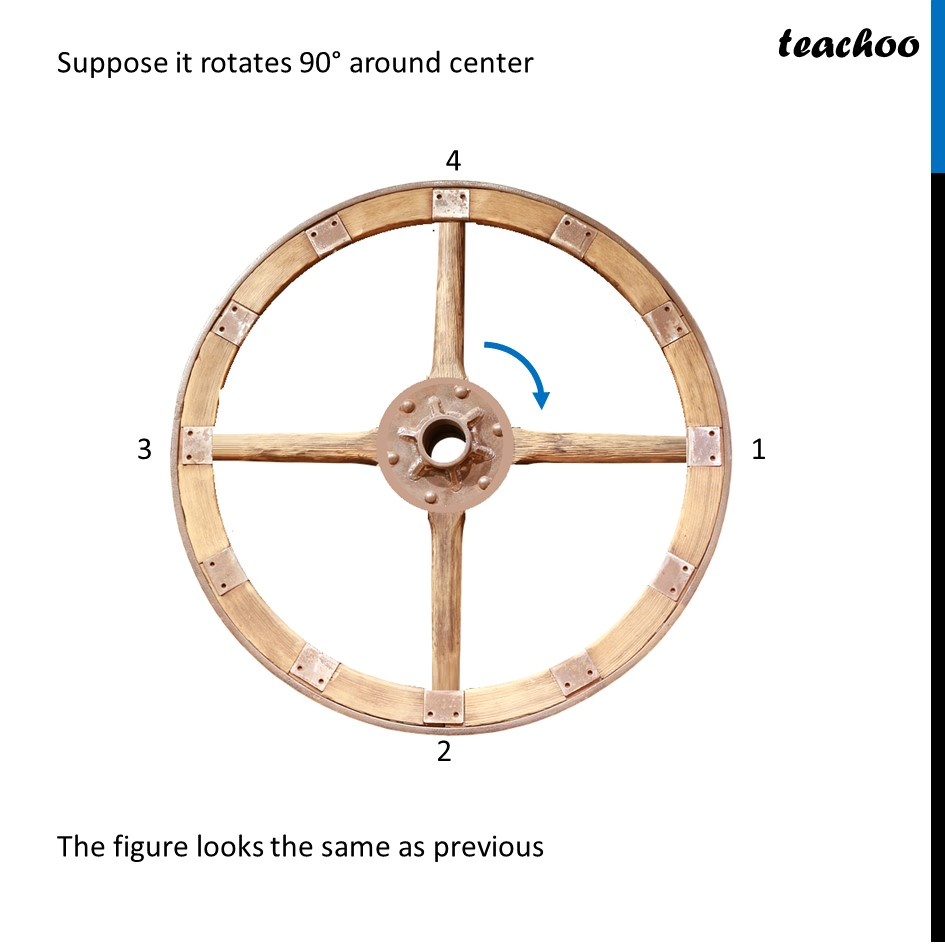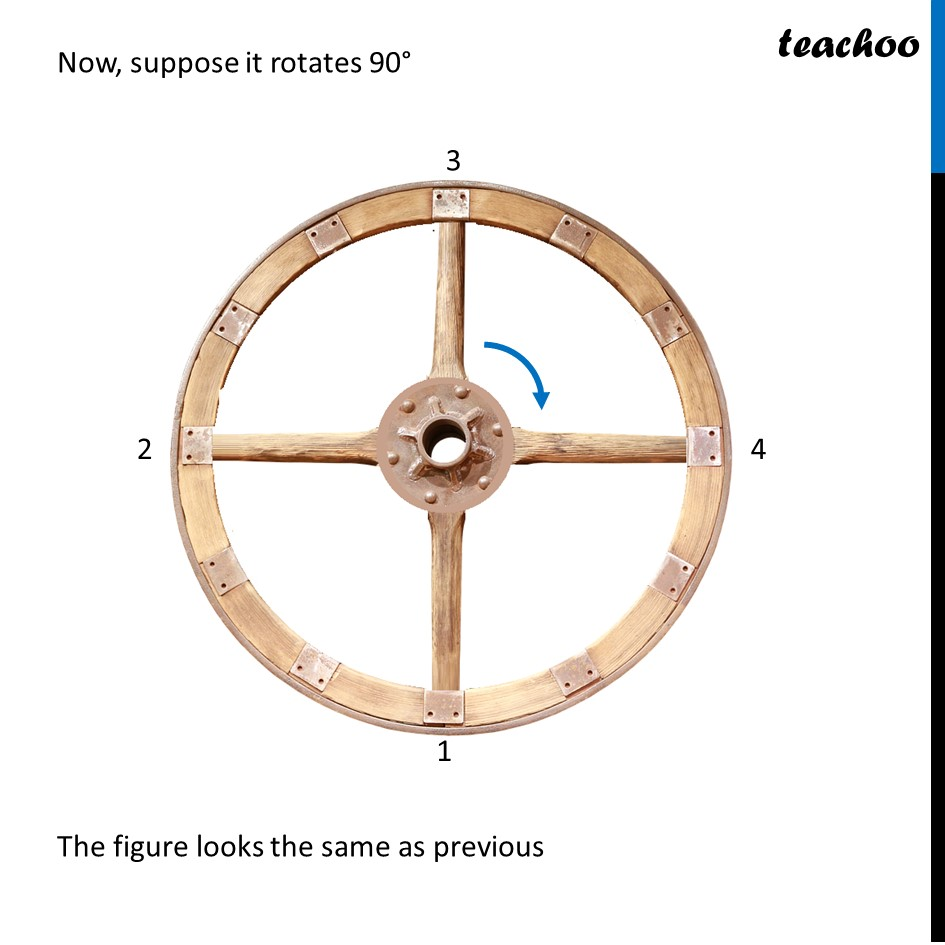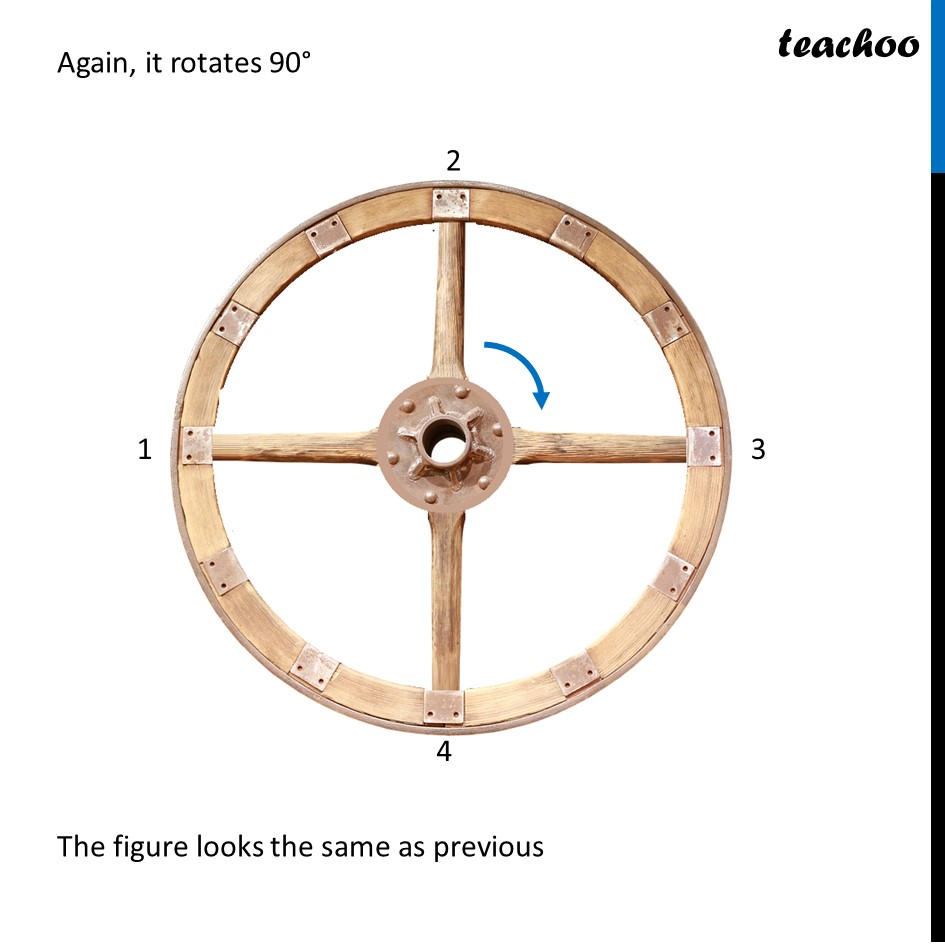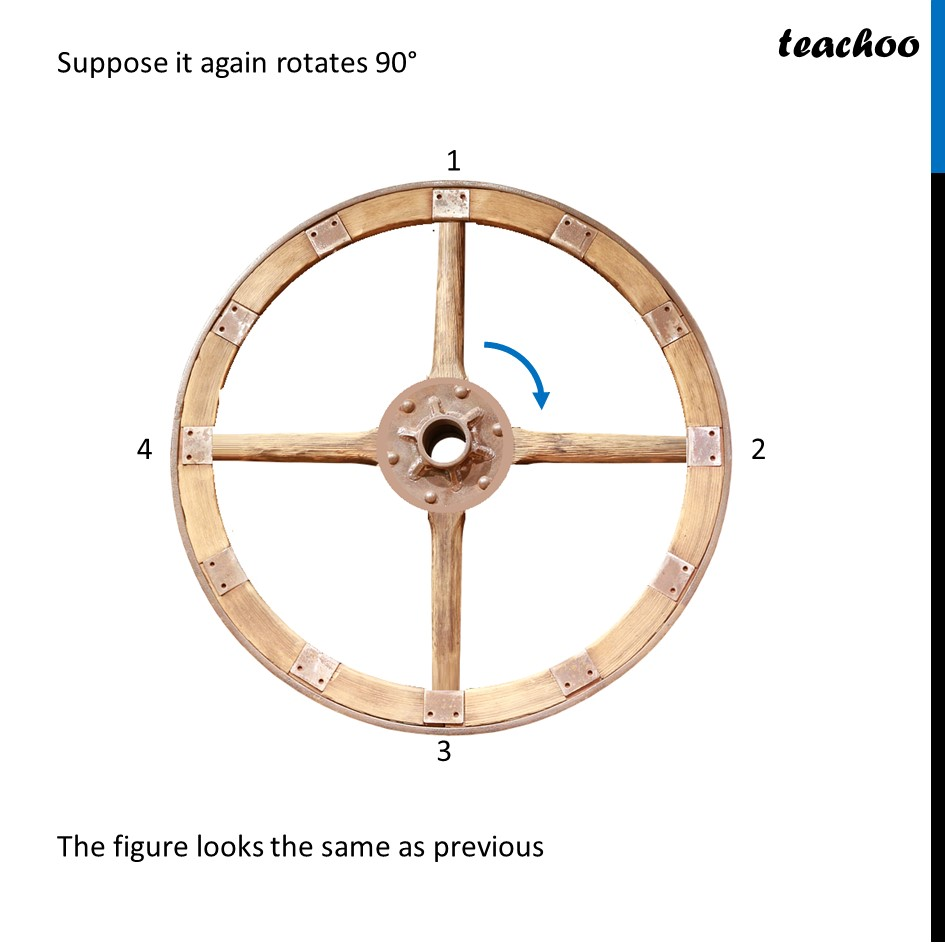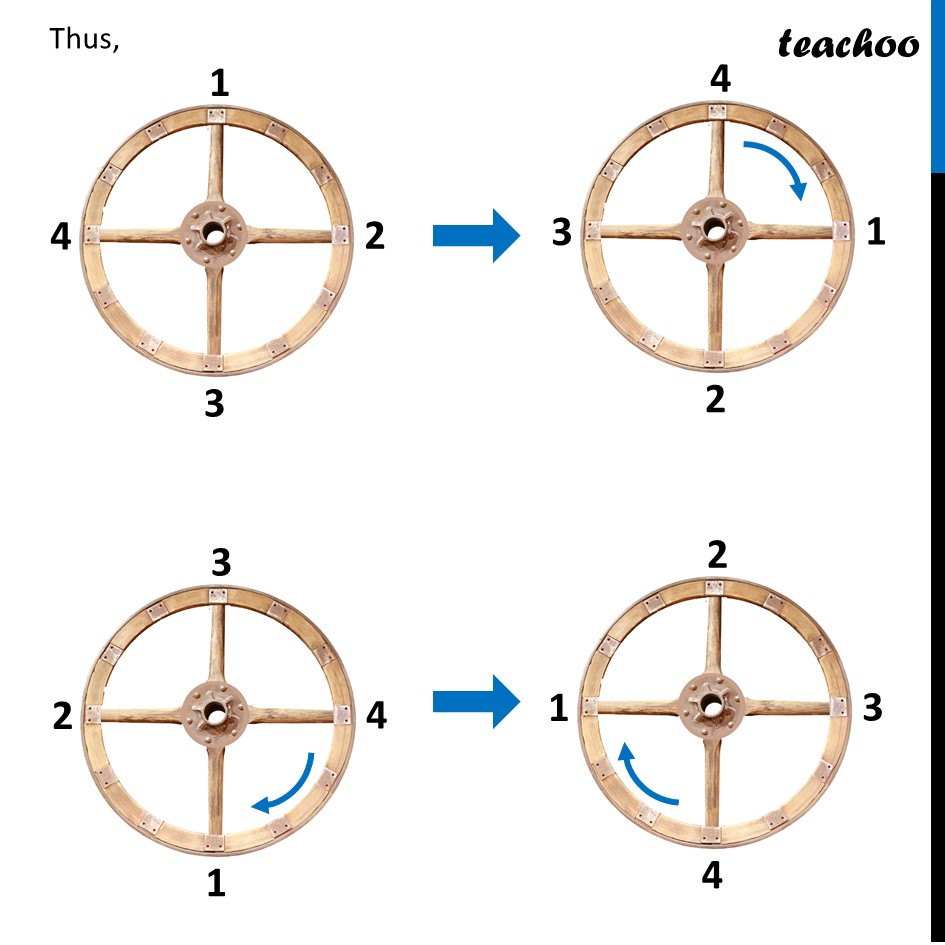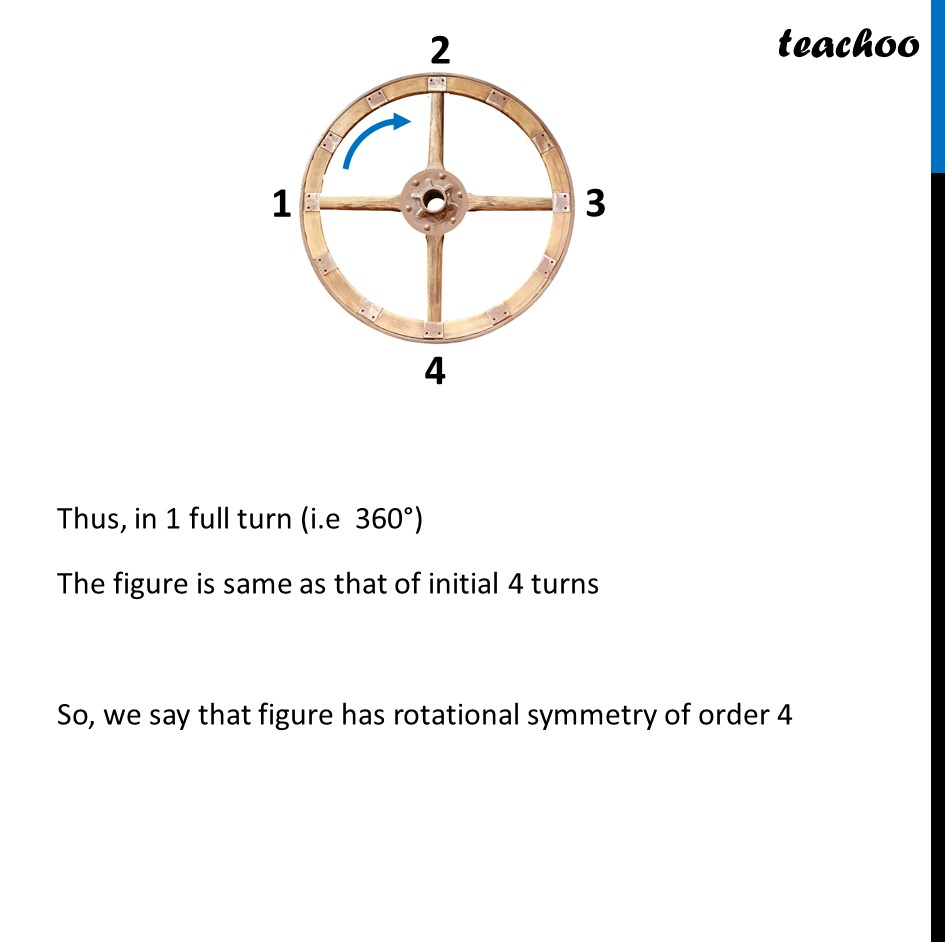### Transcript

Rotational Symmetry - WheelFor a wheel, Suppose it rotates 90° around center The figure looks the same as previous Now, suppose it rotates 90° The figure looks the same as previous Now, suppose it rotates 90° The figure looks the same as previous Again, it rotates 90° The figure looks the same as previous Suppose it again rotates 90° The figure looks the same as previous Thus, Thus, in 1 full turn (i.e 360°) The figure is same as that of initial 4 turns So, we say that figure has rotational symmetry of order 4